# AP Calculus AB : Understanding the derivative of exponents

## Example Questions

### Example Question #1 : Understanding Derivatives Of Exponents

Find the derivative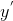for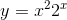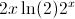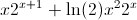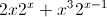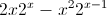Explanation:

The derivative must be computed using the product rule.  Because the derivative ofbrings adown as a coefficient, it can be combined with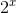to give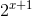### Example Question #3 : Specific Derivatives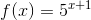What is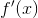?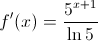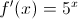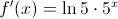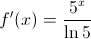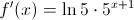Explanation: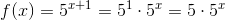Therefore,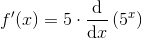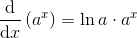for any real, so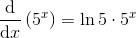, and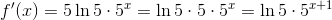### Example Question #4 : Specific Derivatives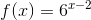What is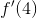?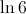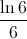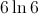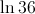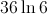Explanation: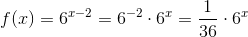Therefore,for any positive, so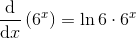, and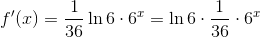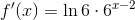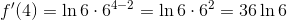### Example Question #2 : Specific Derivatives

Give the instantaneous rate of change of the function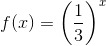at.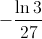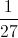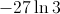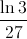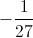Explanation:

The instantaneous rate of change ofat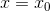is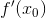, so we will findand evaluate it at.for any positive, so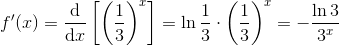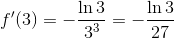### All AP Calculus AB Resources